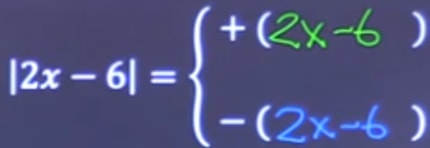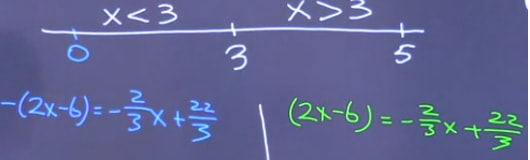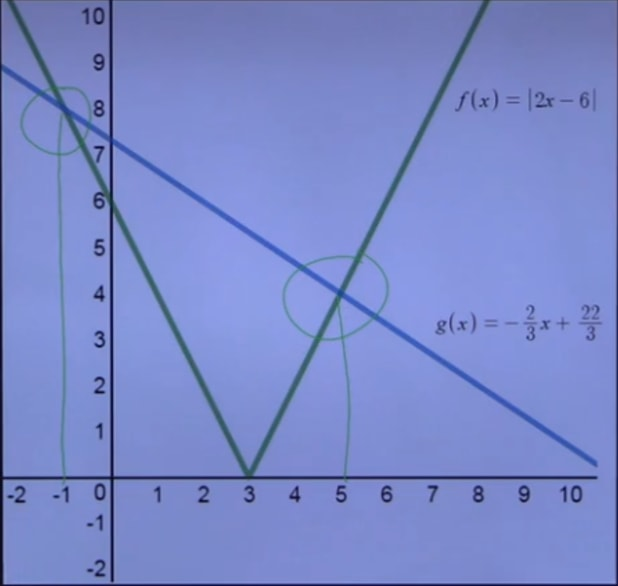# Solving absolute value equations

## What is Absolute Value?

Before we get into solving equations containing absolute values, we must first have a firm grasp at what "absolute value" actually means. See our article on the absolute value function for more information, as well. Also, before reading further, be sure to refresh your memory on how to solve polynomial equations, solving quadratic equations by factoring, and solving quadratic equations by completing the square.

In the most basic sense, the absolute value of something is simply just the "positive" version of it. Take for example the absolute value of -8, which we express as |-8| (the long bars on either side are the notation for absolute value), in this case, the absolute value of -8 is simply just 8. Again, all we need to do in this case is make -8 positive, and thus we have simply 8. In the case of equations, as we'll see next, the process of evaluating these absolute value expressions is just the same!

## Absolute Value Equations:

Now that we understand absolute values and their importance, let's take a closer look at equations with absolute value. Absolute value equations or expressions are evaluated the same way as we did with |-8| above. Let's look at a couple of small examples to make sure we have a good grasp of the concept of absolute value.

i) $|2x - 8| = 2x + 8$

ii) $|-48x^{2} + 4x - 8x^{3}| = 48x^{2} + 4x + 8x^{3}$

As you can see, just like before, all we are doing is making every term in the equation positive. In other words, we are taking the absolute value. This process is the same for all algebraic expressions. However, where things are different – and far more tricky – is when we try solving absolute value equations.

## Solving Absolute Value Equations:

Learning how to solve absolute value equations is actually a simple task – all we have to do is practice a bit first! As always, the best way to do so is to take a look at an example problem.

When it comes to solving absolute value equations, there are several methods. The first, and arguably the easiest, is to solve algebraically. We will go over the algebraic technique in this lesson.

Example 1:

Solve the absolute value equation algebraically, and then verify using the absolute value graph.

$|2x - 6| = - \frac{2x}{3}+ \frac{22}{3}$

STEP 1: Express the Absolute Value as a Piecewise Function

Before we solve the equation, it is very important to recognize the tricky difference that occurs when solving absolute value equations – we can't just consider the positive value. Because we are solving the equation, we don't know the value x. The value for x can be positive or negative, and thus we must account for both cases. To accomplish this, we create what is known as a "piecewise" function.

When we express |2x-6| as a piecewise function, we end up with two cases – the negative piece and the positive piece:

+(2x - 6)

-(2x - 6)Two expression of an absolute value of function

STEP 2: Solve Each Case

Next, all we need to do is make each case equal to the right side of the given equation.

i) Negative Piece:

$-(2x - 6) = - \frac{2x}{3} + \frac{22}{3}$

$3(-2x + 6 = - \frac{2x}{3} + \frac{22}{3})$

$-6x + 18 = -2x + 22$

$-4 = 4x$

$x = -1$

ii) Positive Piece:

$(2x - 6) = - \frac{2x}{3} + \frac{22}{3}$

$3((2x - 6) = - \frac{2x}{3} + \frac{22}{3})$

$6x - 18 = -2x + 22$

$8x = 40$

$x = 5$

We are then left with x = -1 and x = 5 as potential solutions.

STEP 3: Check The Answer

For our third and final step, all we need to do is check that our above solutions are correct. To do this, there are three main methods. We will go over all of them.

1) Number Line

This first strategy is arguably the most difficult. The latter two are much easier, and are recommended. Nevertheless, we will still go over this method. First, solve for x in our absolute value expression to find out at what point on the function is the vertex.

2x - 6 = 0

$x = \frac{6}{2} = 3$

Thus, our "vertex" is 3. On a number line, this gives us regions on the number line that are x < 3 and x > 3.Vertex of 3

In the region x < 3, x can be any number that is smaller than 3 to |2x - 6|. If the result is a negative number, we pick the negative piece in the function. In the region x > 3, x can be any number that is greater than 3 to |2x - 6|. If the result is a positive number, we pick the positive piece in the function.

If we look at our solutions again on this number line, we end up with:Solution on the number line

Now, let's evaluate our solutions to see if they are valid.

Negative Piece:

$-(2x - 6) = - \frac{2x}{3} + \frac{22}{3}$

x = -1

Compare x = -1 to x < 3. -1 < 3 is true. So, we retain the solution.

Positive Piece:

$(2x - 6) = - \frac{2x}{3} + \frac{22}{3}$

x = 5

Compare x = 5 to x > 3. 5 > 3 is true. So, we retain the solution.

2) Left Side = Right Side

In this method, all we have to do is plug in our potential solutions of x = -1 and x = 5 into the equation and see if the left side equals the right side. Let's go ahead and try each solution with this technique.

$x = -1$

$|2x - 6| = - \frac{2x}{3} + \frac{22}{3}$

$|2(-1) - 6| = - \frac{2(-1)}{3} + \frac{22}{3}$

$|-8| = \frac{2}{3} + \frac{22}{3}$

$8 = \frac{24}{3}$

$8 = 8$

Therefore, x = -1 is a valid solution

$x = 5$

$|2x - 6| = - \frac{2x}{3} + \frac{22}{3}$

$|2(5) - 6| = - \frac{2(5)}{3} + \frac{22}{3}$

$|4| = \frac{-10}{3} + \frac{22}{3}$

$4 = \frac{12}{3}$

$4 = 4$

Therefore, x = 5 is a valid solution

3) Graphing

For easy-to-graph functions, all we need to do is graph both the left and right equations, and see where they intersect. If they intersect at our solved solutions, our solutions are valid. Below is a graph of this example.Graphing to see if they intersect

And that's all there is to it! For further, related study, see how articles on how to solve radical equations and solving rational equations. Also, for an excellent tool to check your work, check out this link here.

### Solving absolute value equations

An absolute value or modulus of a real number is always positive. Therefore, when solving absolute value equations, we need to establish two cases for each equation. One case is to set the quantity inside the absolute value notation as positive; whereas another case is to set the value as negative.

#### Lessons

• 1.
Solving Equations with One Absolute Value
Solve each absolute value equation:
i) algebraically
ii) verify the solution graphically
a)
$\left| {2x - 6} \right| = - \frac{2}{3}x + \frac{{22}}{3}$

b)
$\left| {3{x} + 6} \right| = x - 4$

c)
$\left| {{x^2} - 25} \right| = 24$

• 2.
Solving Equations with Two Absolute Values
Solve each absolute value equation:
i) algebraically
ii) verify the solution graphically
a)
$\left| {4 + {x}} \right| - \left| {3{x} - 2} \right| = 2$

b)
$\left| {{x} + 1} \right| = \left| {6 - 3{x}} \right|$